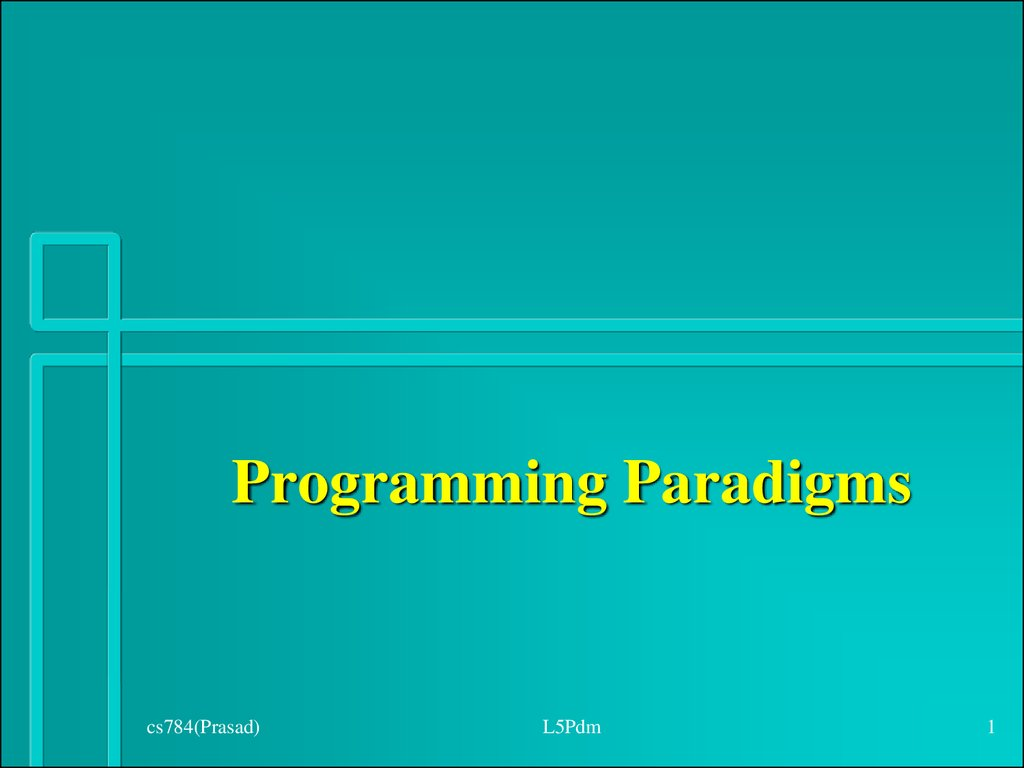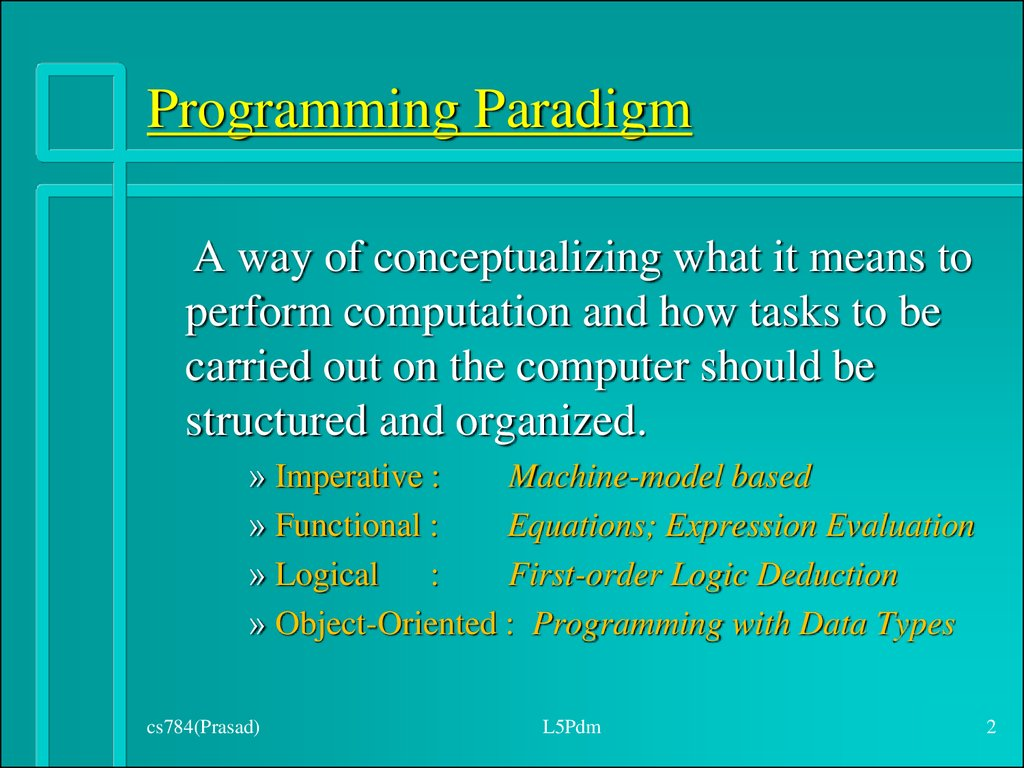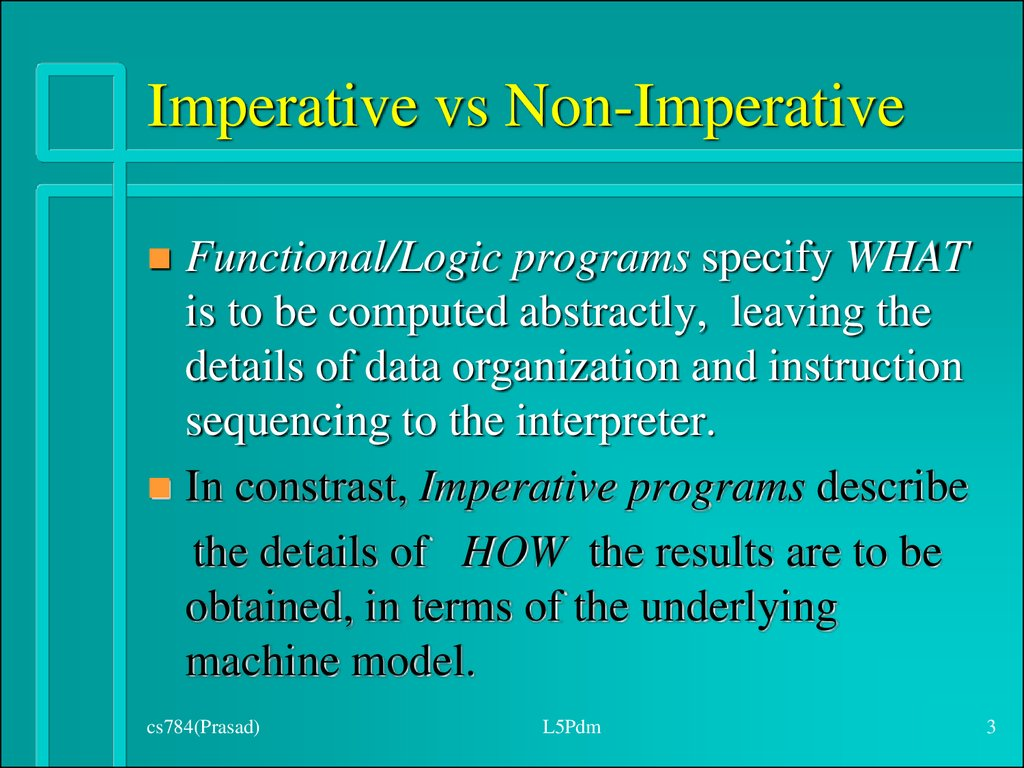## 1.

L5Pdm
1

A way of conceptualizing what it means to
perform computation and how tasks to be
carried out on the computer should be
structured and organized.
» Imperative :
Machine-model based
» Functional :
Equations; Expression Evaluation
» Logical :
First-order Logic Deduction
» Object-Oriented : Programming with Data Types
L5Pdm
2

## 3. Imperative vs Non-Imperative

Functional/Logic programs specify WHAT
is to be computed abstractly, leaving the
details of data organization and instruction
sequencing to the interpreter.
In constrast, Imperative programs describe
the details of HOW the results are to be
obtained, in terms of the underlying
machine model.
L5Pdm
3

## 4. Illustrative Example

Expression (to be computed) : a + b + c
Recipe for Computation:
– Intermediate Code
» T := a + b;
T := T + c;
– Accumulator Machine
– Stack Machine
L5Pdm
4

## 5. Imperative vs Non-Imperative

Functional/Logic style clearly separates
WHAT aspects of a program (programmers’
responsibility) from the HOW aspects
(implementation decisions).
An Imperative program contains both the
specification and the implementation
details, inseparably inter-twined.
L5Pdm
5

## 6. Procedural vs Functional

Program: a sequence
of instructions for a
von Neumann m/c.
Computation by
instruction execution.
Iteration.
Modifiable or
updateable variables.
L5Pdm
Program: a collection
of function definitions
(m/c independent).
Computation by term
rewriting.
Recursion.
Assign-only-once
variables.
6

## 7. Functional Style : Illustration

Definition : Equations
sum(0) = 0
sum(n) = n + sum(n-1)
Computation : Substituition and Replacement
sum(2)
=
2 + sum (2-1)
=

=
3
L5Pdm
7

Imperative Style
i := 0; sum := 0;
while (i < n) do
i := i + 1;
sum := sum + i
end;
– Storage efficient
Functional Style
func sum(i:int) : int;
if i = 0
then 0
else i + sum(i-1)
end;
– No Side-effect
L5Pdm
8

i
0 1 2 3 ...
sum
0 1 3 6 ...
i1
6
3
i2
2
3
i3
1
1
... L5Pdm
sum1
sum2
sum3
9

## 10. Bridging the Gap

Tail recursive programs can be auomatically
optimized for space by translating them into
equivalent while-loops.
func sum(i : int, r : int) : int;
if i = 0 then r
else sum(i-1, n+r)
end
– Scheme does not have loops.
L5Pdm
10

## 11. Analogy: Styles vs Formalisms

Iteration
Regular Expression
Tail-Recursion
Regular Grammar
General Recursion
Context-free Grammar
L5Pdm
11

Integrates Data and Control Structures
edge(a,b).
edge(a,c).
edge(c,a).
path(X,X).
path(X,Y) :- edge(X,Y).
path(X,Y) :- edge(X,Z), path(Z,Y).
L5Pdm
12

## 13. Declarative Programming

A logic program defines a set of relations.
This “knowledge” can be used in various
ways by the interpreter to solve different
queries.
In contrast, the programs in other languages
make explicit HOW the “declarative
knowledge” is used to solve the query.
L5Pdm
13

## 14. Append in Prolog

append([], L, L).
append([ H | T ], L, [ H | R ]) :append(T, L, R).
» “.” and “:-” are logical connectives that stand for
“and” and “if” respectively.
Uses pattern matching.
» “[]” and “|” stand for empty list and cons operation.
L5Pdm
14

## 15. Different Kinds of Queries

Verification
– sig: list x list x list
» append(, [2,3], [1,2,3]).
Concatenation
– sig: list x list -> list
» append(, [2,3], R).
L5Pdm
15

## 16. More Queries

Constraint solving
– sig: list x list -> list
» append( R, [2,3], [1,2,3]).
– sig: list -> list x list
» append(A, B, [1,2,3]).
Generation
– sig: -> list x list x list
» append(X, Y, Z).
L5Pdm
16

## 17.

Executable specification
Knowledge
Representation
Problem Solving in AI
(i) Search
(ii) Divide and Conquer
Theorem
Proving
efficiency
unification
mechanization
Attribute Grammars
/ Compilers (DCGs)
expressiveness
Relational
Databases
L1LP
declarativeness
Programming
Languages
17

## 18. Object-Oriented Style

Programming with Abstract Data Types
Basic Program Unit: Class
» Abstraction enforced by encapsulation.
Basic Run-time Unit: Object
– Instance of a class.
» Has an associated state.
L5Pdm
18

## 19. Procedural vs Object-Oriented

Emphasis on
procedural abstraction.
Top-down design;
Step-wise refinement.
Suited for
programming in the
small.
L5Pdm
Emphasis on data
abstraction.
Bottom-up design;
Reusable libraries.
Suited for
programming in the
large.
19

## 20. Integrating Heterogeneous Data

In C, Pascal, etc., use
Union Type / Switch Statement
Variant Record Type / Case Statement
In C++, Java, Eiffel, etc., use
Abstract Classes / Virtual Functions
Interfaces and Classes / Dynamic Binding
L5Pdm
20

Data
– Square
– Square
» side
» side
» area
(= side * side)
– Circle
Classes
– Circle
Operation (area)
» area
– Square
» side * side
– Circle
L5Pdm
21

## 22. Adding a new operation

Data
...
Operation (area)
Operation (perimeter)
– Square
» ...
» perimeter
(= 4 * side)
– Square
– Circle
» 4 * side
» ...
» perimeter
(= 2 * PI * radius)
– Circle
» 2 * PI * radius
Classes
L5Pdm
22

## 23.

Data
– ...
– rectangle
– ...
– rectangle
» length
» width
Classes
» length
» width
» area
(= length * width)
Operation (area)
– ...
– rectangle
» length * width
L5Pdm
23

## 24. Procedural vs Object-Oriented

New operations cause additive changes in
procedural style, but require modifications
to all existing “class modules” in objectoriented style.
changes in object-oriented style, but require
modifications to all “procedure modules”.
L5Pdm
24

## 25. Object-Oriented Concepts

Data Abstraction (specifies behavior)
Encapsulation (controls visibility of names)
Polymorphism (accommodates various
implementations)
Inheritance (facilitates code reuse)
Modularity (relates to unit of compilation)
L5Pdm
25

## 26. Example : Role of interface in decoupling

Client
» Determine the number of elements in a collection.
Suppliers
» Collections : Vector, String, List, Set, Array, etc
Procedual Style
» A client is responsible for invoking appropriate
supplier function for determining the size.
OOP Style
» Suppliers are responsible for conforming to the
standard interface required for exporting the size
functionality to a client.
L5Pdm
26

## 27. Client in Scheme

(define (size C)
(cond
( (vector? C)
( (pair? C)
( (string? C)
( else
))
(size
(size
(vector-length C) )
(length C) )
(string-length C) )
“size not supported”) )
(vector 1 2 (+ 1 2)))
‘(one “two” 3))
L5Pdm
27

## 28. Suppliers and Client in Java

interface
Collection { int size(); }
class myVector extends Vector
implements Collection {
}
class myString extends String
implements Collection {
public int size() { return length();}
}
class myArray implements Collection {
int[] array;
public int size() {return array.length;}
}
Collection c = new